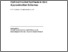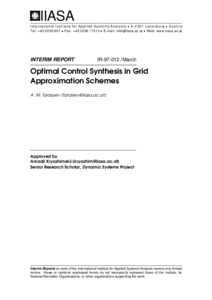# Optimal Control Synthesis in Grid Approximation Schemes

Tarasyev, A.M. (1997). Optimal Control Synthesis in Grid Approximation Schemes. IIASA Interim Report. IIASA, Laxenburg, Austria: IR-97-012Preview
Text
IR-97-012.pdf

## Abstract

Grid approximation schemes for constructing value functions and optimal feedbacks in problems of guaranteed control are proposed via a theory of generalized (minimax, viscosity) solutions of Hamilton-Jacobi equations. Value functions in optimal control problems are usually nondifferentiable and corresponding feedbacks have the discontinuing switching character. Therefore, constructions of generalized gradients for local hulls of different types are used in finite difference operators which approximate value functions. Optimal feedbacks are synthesized by external shift in the direction of the generalized gradients. Both problems of constructing the value function and control synthesis are solved simultaneously in the unique grid scheme. The interpolation problem is analyzed for grid values of optimal feedbacks. Questions of correlating spatial and temporal meshes are examined. Significance of quasiconvexity properties is clarified for the linear dependence of space-time grids.

The proposed grid schemes for solving optimal guaranteed control problems can be applied for models arising in mechanics, mathematical economics, differential and evolutionary games.

Item Type: Monograph (IIASA Interim Report) Dynamic Systems (DYN) IIASA Import 15 Jan 2016 02:09 27 Aug 2021 17:16 http://pure.iiasa.ac.at/5279View Item